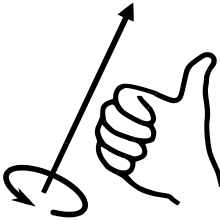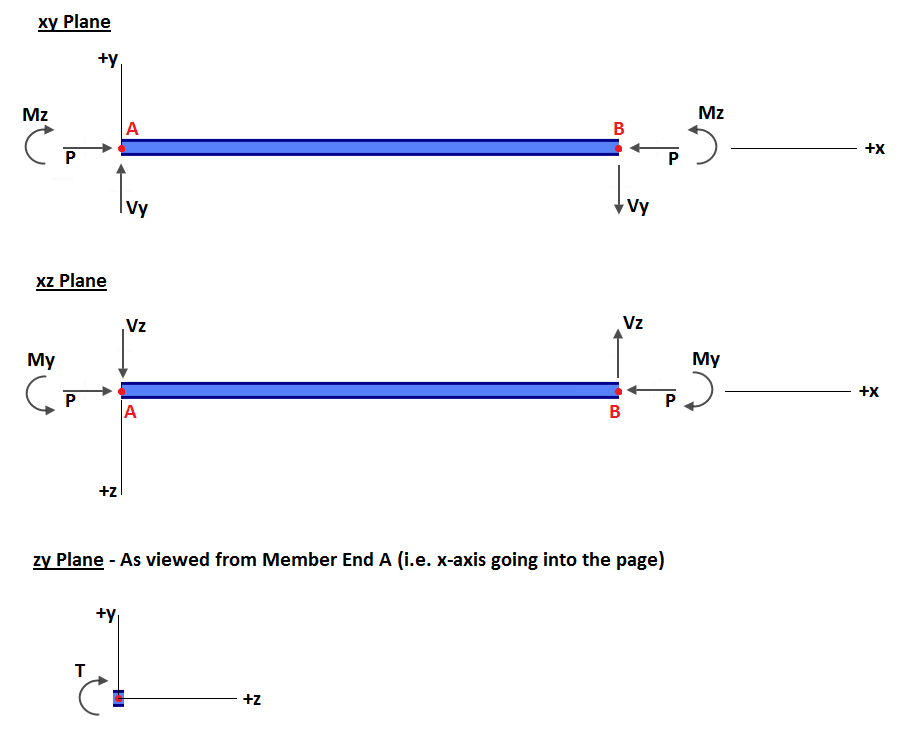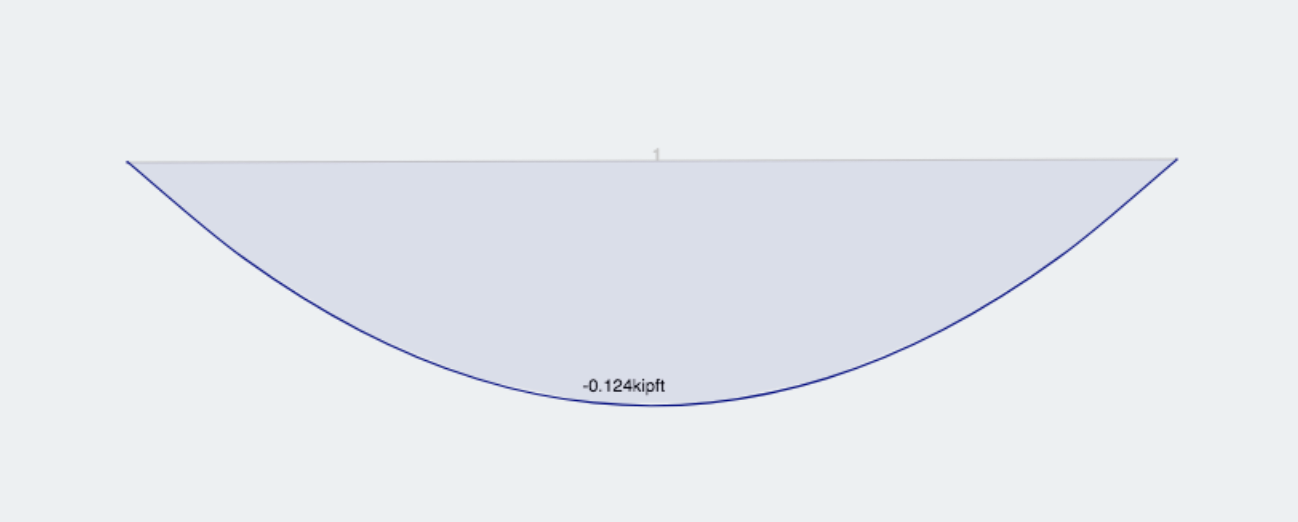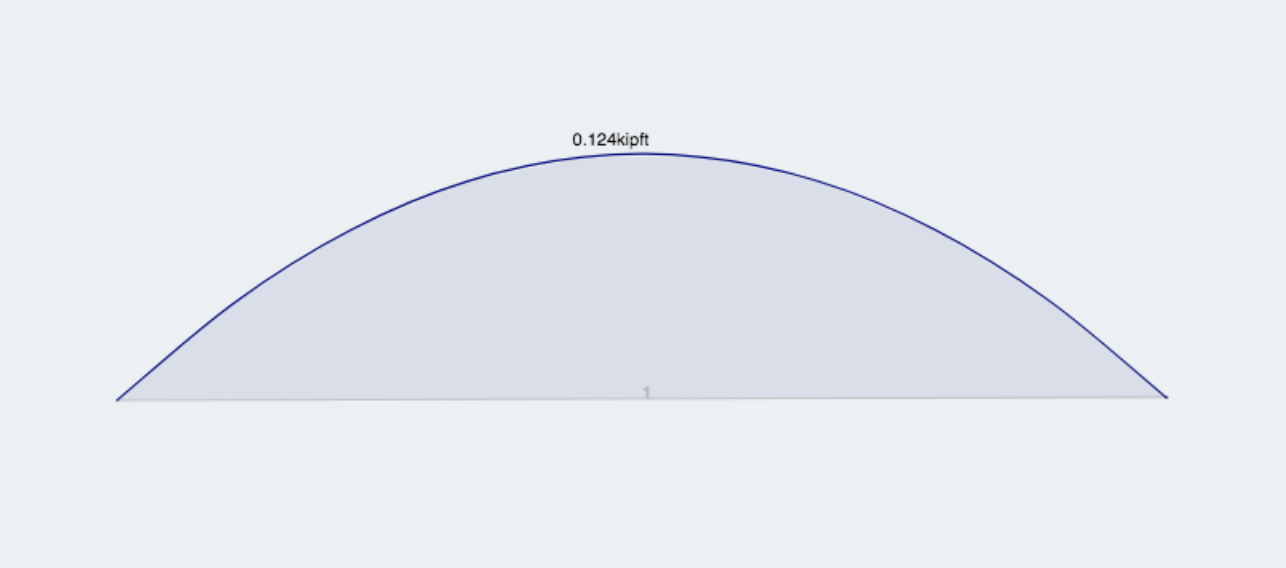Documentation [Deprecated]
SkyCiv Documentation

Getting Started

Section Builder
Operations
Built-Up and Composite Sections
Custom Shapes

Member Design
General
Member Design Modules
Code Verification

RC Design
General
Code Verification

# Sign Conventions

### General

Applied loads, displacements and reactions are positive when they act along or about the positive axis direction. For rotations (like moments) the positive direction is with respect to the RIGHT HAND RULE:Be wary of the axes which are used to define loads. By default, loads are along the global axes however distributed loads can be defined with respect to the local member axis. Similarly, plate pressures can be defined globally or locally to the plate. Also remember that the local axes of members and plates can be viewed and toggled from the Visibility Settings.

### Member ActionsIn the diagram above:

• P = Axial Force
• T = Torsional Force (i.e. moment about member's x-axis)
• Vy = Shear Force in member's y-axis
• Vz = Shear Force in member's z-axis
• My = Bending Moment in member's y-axis
• Mz = Bending Moment in member's z-axis

The diagram above can be summarized as follows:

• Positive Axial Force (P): Compression
• Positive Moment (M): Compression on the positive axis side of the member
• Positive Shear (V): Node A of the member translates in the positive axis with respect to Node B.
• Positive Torsion (T): Node B rotates clockwise when viewed from the Node A end. Or Node A of the member rotates counter-clockwise when viewed from the Node B end. Both instances conform to the right-hand rule.

NOTE: There is a setting in Settings called "Reverse BMD Sign" which reverses the sign convention of the bending moment diagrams to suit whichever convention you're most familiar with.

### Simple Example

By default, a simple downward distributed load with two point loads will display the following bending moment diagram:If the "Reverse BMD Sign" setting is toggled on, the exact same model would produce the following bending moment diagram:Coming soon..